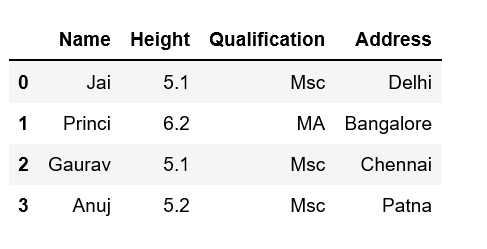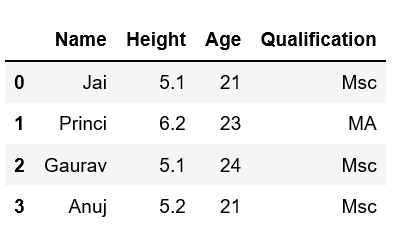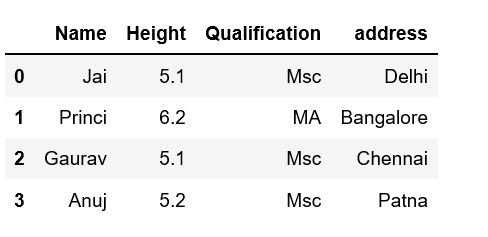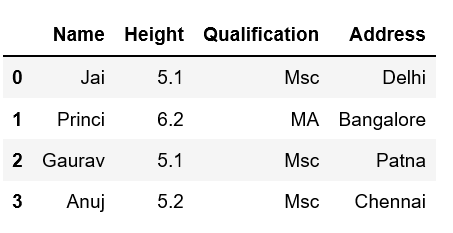Open in App
Not now

# Adding new column to existing DataFrame in Pandas

• Difficulty Level : Easy
• Last Updated : 11 Jan, 2023

Let’s discuss how to add new columns to the existing DataFrame in Pandas. There are multiple ways we can do this task.

Method #1: By declaring a new list as a column.

## Python3

 `# Import pandas package ` `import` `pandas as pd ` ` `  `# Define a dictionary containing Students data ` `data ``=` `{``'Name'``: [``'Jai'``, ``'Princi'``, ``'Gaurav'``, ``'Anuj'``], ` `        ``'Height'``: [``5.1``, ``6.2``, ``5.1``, ``5.2``], ` `        ``'Qualification'``: [``'Msc'``, ``'MA'``, ``'Msc'``, ``'Msc'``]} ` ` `  `# Convert the dictionary into DataFrame ` `df ``=` `pd.DataFrame(data) ` ` `  `# Declare a list that is to be converted into a column ` `address ``=` `[``'Delhi'``, ``'Bangalore'``, ``'Chennai'``, ``'Patna'``] ` ` `  `# Using 'Address' as the column name ` `# and equating it to the list ` `df[``'Address'``] ``=` `address ` ` `  `# Observe the result ` `print``(df) `

Output:Note that the length of your list should match the length of the index column otherwise it will show an error.

Method #2: By using DataFrame.insert()

It gives the freedom to add a column at any position we like and not just at the end. It also provides different options for inserting the column values.

Example

## Python3

 `# Import pandas package  ` `import` `pandas as pd ` ` `  `# Define a dictionary containing Students data ` `data ``=` `{``'Name'``: [``'Jai'``, ``'Princi'``, ``'Gaurav'``, ``'Anuj'``], ` `        ``'Height'``: [``5.1``, ``6.2``, ``5.1``, ``5.2``], ` `        ``'Qualification'``: [``'Msc'``, ``'MA'``, ``'Msc'``, ``'Msc'``]} ` ` `  `# Convert the dictionary into DataFrame ` `df ``=` `pd.DataFrame(data) ` ` `  `# Using DataFrame.insert() to add a column ` `df.insert(``2``, ``"Age"``, [``21``, ``23``, ``24``, ``21``], ``True``) ` ` `  `# Observe the result ` `print``(df) `

Output:Method #3: Using Dataframe.assign() method

This method will create a new dataframe with a new column added to the old dataframe.

Example

## Python3

 `# Import pandas package ` `import` `pandas as pd ` ` `  `# Define a dictionary containing Students data ` `data ``=` `{``'Name'``: [``'Jai'``, ``'Princi'``, ``'Gaurav'``, ``'Anuj'``], ` `        ``'Height'``: [``5.1``, ``6.2``, ``5.1``, ``5.2``], ` `        ``'Qualification'``: [``'Msc'``, ``'MA'``, ``'Msc'``, ``'Msc'``]} ` ` `  ` `  `# Convert the dictionary into DataFrame ` `df ``=` `pd.DataFrame(data) ` ` `  `# Using 'Address' as the column name and equating it to the list ` `df2 ``=` `df.assign(address``=``[``'Delhi'``, ``'Bangalore'``, ``'Chennai'``, ``'Patna'``]) ` ` `  `# Observe the result ` `print``(df2) `

Output:Method #4: By using a dictionary

We can use a Python dictionary to add a new column in pandas DataFrame. Use an existing column as the key values and their respective values will be the values for a new column.

Example

## Python3

 `# Import pandas package ` `import` `pandas as pd ` ` `  `# Define a dictionary containing Students data ` `data ``=` `{``'Name'``: [``'Jai'``, ``'Princi'``, ``'Gaurav'``, ``'Anuj'``], ` `        ``'Height'``: [``5.1``, ``6.2``, ``5.1``, ``5.2``], ` `        ``'Qualification'``: [``'Msc'``, ``'MA'``, ``'Msc'``, ``'Msc'``]} ` ` `  `# Define a dictionary with key values of ` `# an existing column and their respective ` `# value pairs as the # values for our new column. ` `address ``=` `{``'Delhi'``: ``'Jai'``, ``'Bangalore'``: ``'Princi'``, ` `           ``'Patna'``: ``'Gaurav'``, ``'Chennai'``: ``'Anuj'``} ` ` `  `# Convert the dictionary into DataFrame ` `df ``=` `pd.DataFrame(data) ` ` `  `# Provide 'Address' as the column name ` `df[``'Address'``] ``=` `address ` ` `  `# Observe the output ` `print``(df) `

Output:My Personal Notes arrow_drop_up
Related Articles